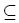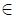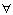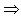Competitions

# The Bottom of a Graph

Time limit 1 second
Memory limit 64 MiBWe will use the following (standard) definitions from graph theory. Let V be a nonempty and finite set, its elements being called vertices (or nodes). Let EV×V , its elements being called edges. Then G = (V,E) is called a directed graph.Let n be a positive integer, and let p = (e1, ..., en) be a sequence of length n of edges eiE such that ei = (vi, vi+1) for a sequence of vertices (v1, ..., vn+1). Then p is called a path from vertex v1 to vertex vn+1 in G and we say that vn+1 is reachable from v1, writing (v1 vn+1). Here are some new definitions. A node v in a graph G = (V, E) is called a sink, if for every node w in G that is reachable from v, v is also reachable from w. The bottom of a graph is the

subset of all nodes that are sinks, i.e.,bottom(G) = {v V | (w V )v w w v}.

## Input dataThe input contains several test cases, each of which corresponds to a directed graph G. Each test case starts with an integer number v, denoting the number of vertices of G = (V, E), where the vertices will be identified by the integer numbers in the set V = {1, ..., v}. You may assume that 1v5000. That is followed by a non-negative integer eand then e pairs of vertex identifiers v1, w1, ..., ve, we with the meaning that (vi, wi)E. There are no edges other than specified by these pairs. The last test case is followed by a zero.

## Output data

For each test case output the bottom of the specified graph on a single line. To this end, print the numbers of all nodes that are sinks in sorted order separated by a single space character. If the bottom is empty, print an empty line.

## Examples

Input example #1
3 3
1 3 2 3 3 1
2 1
1 2
0

Output example #1
1 3
2